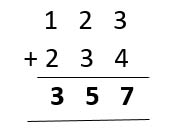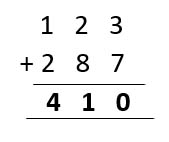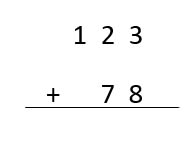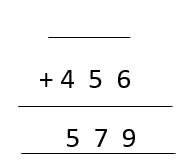• These worksheets are designed to help your child practice and improve their 3-digit addition skills in various ways. Whether your child needs to review the basics of 3-digit addition or wants to challenge themselves with word problems and puzzles, we have something for everyone.

## What are 3-digit addition worksheets?

3-digit addition worksheets are printable math activities that involve adding numbers with three digits. For example, 123 + 456 = 579 is a 3-digit addition problem.

These worksheets can have different formats and levels of difficulty, depending on the skills and goals of the students. Some common types of 3-digit addition worksheets are:

• Adding without regrouping (or carrying): This means that the sum of each column is less than 10. For example;• Adding with and without regrouping: This means that some columns require regrouping, and some don't. For example, 123 + 456 = 579. In this case, you only have to regroup in the tens column.

• Adding with regrouping (or carrying): This means that the sum of one or more columns is greater than 10. For example, 123 + 287 = 410. In this case, you must regroup (or carry) the extra digit to the next column.In the ones column, 3 + 7 = 10. You write down 0 and carry the 1 to the tens column.

Then, add the tens column: 1 + 2 + 8 = 11.

You write down 1 and carry the 1 to the hundreds column.

Finally, you add the hundreds column: 1 + 1 + 2 = 4.

You write down the final answer: 410.

• ### BROWSE THE WEBSITE

#### Start practice on Second Grade here

MathSkills4Kids 3-digit addition worksheets for 2nd graders is a fantastic resource designed to build everlasting math skills in your early math learners.

These worksheets are a great way to practice and reinforce 3-digit addition skills in a fun and engaging way. They can also help your child develop their number sense, mental math, and problem-solving abilities.

Here are some of the skills you'll find in MathSkills4Kids worksheets:

• Adding multiple of 100: These worksheets help students practice adding numbers that end with two zeros, such as 200 + 300 = 500.

This is a good way to introduce the concept of place value and how it affects addition.

• Adding 3-digit numbers without and with regrouping using models: These worksheets help students understand how to add 3-digit numbers by breaking them down into hundreds, tens, and ones.

They also teach students how to regroup or carry over when the sum of two digits is more than 10. For example, in 123 + 456 = 579, we need to regroup the ones and tens as follows: (3 + 6) = (9 + 0),     (2 + 5 + 0) = (7 + 0),     (1 + 4 + 0) = (5 + 0).

These worksheets use models such as base-ten blocks, place value charts, or expanded forms to show the steps of adding 3-digit numbers.

• Addition of numbers up to 3-digit: These worksheets help students practice adding any two numbers up to three digits. They can be horizontal or vertical and may or may not require regrouping. For example,    123 + 78 = ___     or• How to complete addition sentences with numbers up to 3-digit: These worksheets help students practice finding the missing number in an addition sentence with numbers up to three digits. For example,    ___ + 456 = 579     or• Writing addition sentences word problems with numbers up to 3-digit: These worksheets help students practice applying their 3-digit addition skills to real-world situations.

They require students to read a word problem, identify the relevant information, write an addition sentence, and solve it. For example,Lisa has collected 123 stamps. Her friend Amy has collected 456 stamps. How many stamps do they have in total?

Addition sentence:    ___ + ___ = ___

Solution:   ___ stamps

• ### How can these worksheets make math practice more enjoyable?

One of the main benefits of using MathSkills4Kids 3-digit addition worksheets for 2nd graders is that they make math practice more enjoyable and engaging for students.

These worksheets are designed with colorful graphics, fun themes, and interactive activities that appeal to young learners and motivate them to practice their 3-digit addition skills.

Students will love solving puzzles, coloring pictures, matching cards, and playing games while learning how to add 3-digit numbers. These worksheets also provide a variety of challenges and levels of difficulty to suit different learning needs and preferences. Students can choose from easy, medium, or hard worksheets depending on their confidence and ability.

They can also progress from simpler to more complex problems as they master each skill.

• ### What skills will students develop by completing these worksheets?

By completing these worksheets, students will develop various skills essential for their math success. These skills include:

• Understanding the place value of 3-digit numbers and how to use it to perform addition.
• Applying different strategies and methods to add 3-digit numbers without and with regrouping, such as using models, base-10 blocks, expanded form, standard algorithm, etc.
• Solving word problems that involve adding 3-digit numbers in various contexts and situations.
• Writing addition sentences that match word problems or models.
• Checking their answers using inverse operations or estimation techniques.
• Developing their mental math skills and fluency in adding 3-digit numbers.
• ### How can parents and teachers use these worksheets to support learning at home or in the classroom?

Parents and teachers can use these worksheets to support learning at home or in the classroom in many ways. Some of the ways are:

• Providing extra practice and reinforcement for students who need more help with 3-digit addition skills.
• Offering enrichment and extension activities for students ready for more challenge and depth with 3-digit addition skills.
• Using these worksheets as homework assignments, classwork tasks, assessment tools, or review materials.
• Incorporating these worksheets into math centers, stations, or rotations where students can work independently or in pairs or groups.
• Creating fun and interactive math games using these worksheets, such as bingo, memory, dominoes, etc.

### Bonus: More online resources to reinforce subtracting within 2-Digits skills for 2nd Graders

Parents and teachers can use these worksheets to support learning at home or in the classroom in various ways. Other online resources can complement these worksheets and provide more opportunities for learning and practicing 3-digit addition skills.

Using these resources, students can boost their confidence and competence in adding 3-digit numbers.

With these additional resources, you'll be able to improve your 3-digit addition skills in no time. Have fun and keep practicing!

Thank you for sharing the links of MathSkills4Kids.com with your loved ones. Your choice is greatly appreciated.

To conclude, we want to reiterate that MathSkills4Kids 3-digit addition worksheets for 2nd graders are an excellent resource for helping students master this important math skill. These worksheets are designed to make math practice more enjoyable, engaging, and effective for students.

They also help students develop various skills essential for their math success.

•